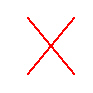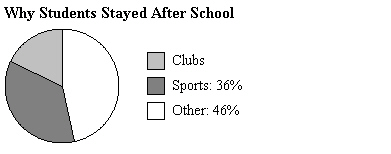Name:    Chapter 4 Test

Multiple Choice
Identify the choice that best completes the statement or answers the question.

Estimate the answer to the question using a model.

1.

44 is 80% of what number?
 a. 3520 c. 53 b. 55 d. 54

Write and solve an equation to answer the question.

2.

57 is what percent of 95?
 a. 166.67% c. 60% b. 63% d. 55%

3.

A basketball player made 25 baskets in a season. Of these, 36% were three-point shots. How many three-point shots did the player make?
 a. 16 c. 5 b. 11 d. 9

4.

A club raised 96% of its goal for a charity. The club raised \$1056. What was the goal?
 a. \$1022.76 c. \$1098 b. \$1100 d. \$1013.76

Find the new amount.

5.

50 feet increased by 184%
 a. 234 ft c. 92 ft b. 145 ft d. 142 ft

6.

A bike with an original price of \$120 is discounted 20%. What is the sale price?
 a. \$144 c. \$24 b. \$96 d. \$100

7.

A gold chain with an original price of \$120 is on sale for \$66. What is the percent of the discount?
 a. 181% c. 54% b. 45% d. 55%

8.

After a discount of 25%, the sale price of a ride pass at a fun park is \$45. What is the original price?
 a. \$180 c. \$70 b. \$49 d. \$60

Find the cost to store, percent of markup, or selling price.

9.

Cost to store: \$90
Markup: 90%
Selling price:   ?
 a. \$171 c. \$180 b. \$173 d. \$176

Find the annual simple interest rate.

10.

I = \$105, P = \$300, t = 5 years
 a. 7% c. 0.07% b. 35% d. 8%

11.

I = \$56, P = \$350, t = 48 months
 a. 3% c. 16% b. 4% d. 0.04%

12.

A savings account earns 2% annual simple interest. The principal is \$1400. What is the balance after 8 years?
 a. \$224 c. \$23,800 b. \$1624 d. \$22,400

Find the amount paid for the loan.

13.

\$1200 at 9% for 9 years
 a. \$972 c. \$1200 b. \$2183 d. \$2172

Numeric Response

1.

A television with an original price of \$110 is on sale for \$88. What is the percent of the discount?

2.

A principal of \$16,000 is invested at 8% and earns \$8,320.00 simple interest. How long has the money been invested?

An account earns simple interest. (a) Find the interest earned. (b) Find the balance of the account.

1.

\$1150 at 7% for 3 years

2.

Describe and correct the error in finding the simple interest earned on \$400 at 4% for 6 months.I = (400)(0.04)(6) = \$96

Other

1.

Extended Response  The number of students who stayed after school to attend clubs was 27.a.   How many students stayed after school?
b.   How many students stayed for sports?